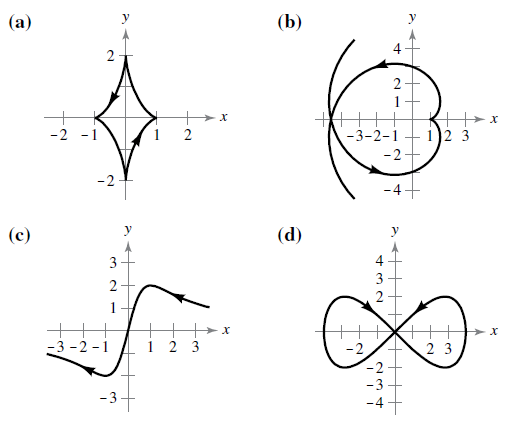Chapter 10.2, Problem 71E

Chapter
Section
Textbook Problem

Matching In Exercises 71–74, match the set of parametric equation with its graphs. [The graphs are labeled (a), (b), (c), and (d).] Explain your reasoning. Lissajous curve : x = 4 cos θ , y = 2 sin 2 θTo determine
To match the curve with the given equation

Explanation

Given: x=4cosθ,y=2sin2θ

Explanation: We plot the graph on Wx Maxima by using the following steps

Step 1: Open Wx Maxima

Step2: Click on the plot section on the toolbar

Step3: Click on the plot 2d section under the plot toolbar

Step 4: Click on parametric which appears after clicking on special button on the right of the bar

Still sussing out bartleby?

Check out a sample textbook solution.

See a sample solution

The Solution to Your Study Problems

Bartleby provides explanations to thousands of textbook problems written by our experts, many with advanced degrees!

Get Started

Factoring Completely Factor the expression completely. 105. 49 4y2

Precalculus: Mathematics for Calculus (Standalone Book)

(t+6)(60)(60t+180)(t+6)2

Applied Calculus for the Managerial, Life, and Social Sciences: A Brief Approach

Solve 3x25x=2

Elementary Technical Mathematics